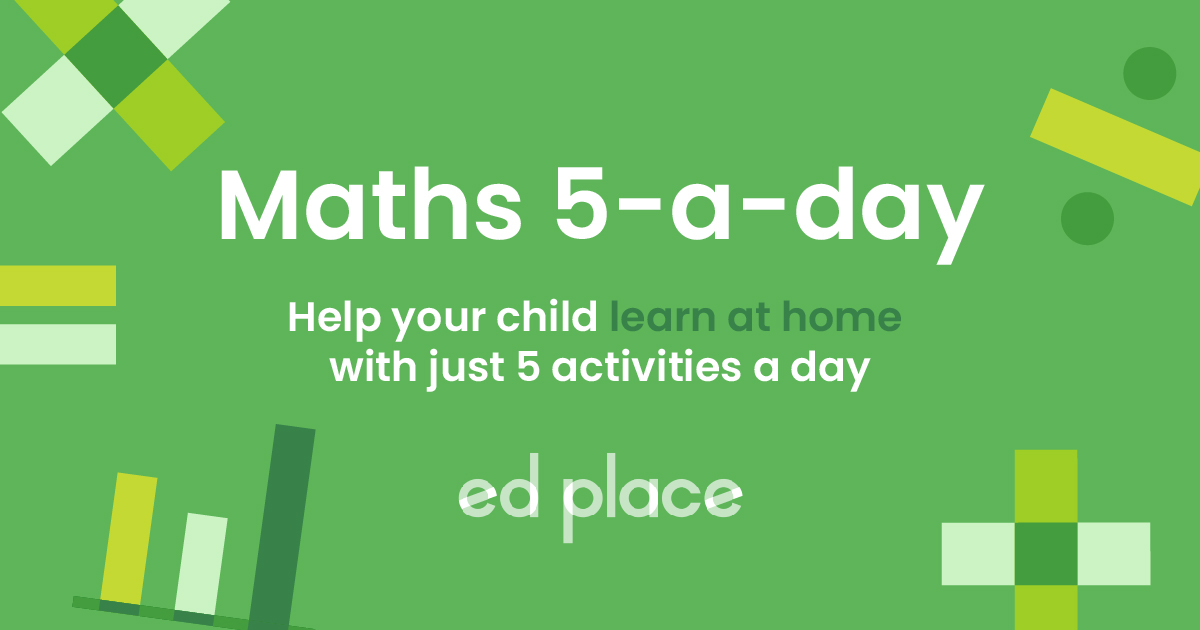# EdPlace's Year 3 and 4 home learning maths lesson: Finding Unit and Non-Unit Fractions of Numbers

Looking for short lessons to keep your child engaged and learning? Our experienced team of teachers have created English, maths and science lessons for the home, so your child can learn no matter where they are.  And, as all activities are self-marked, you really can encourage your child to be an independent learner.

Get them started on the lesson below and then jump into our teacher-created activities to practice what they've learnt. We've recommended five to ensure they feel secure in their knowledge - 5-a-day helps keeps the learning loss at bay (or so we think!).

Are they keen to start practising straight away? Head to the bottom of the page to find the activities.

Now...onto the lesson!

Key Stage 2 Statutory Requirements for Maths
Year 4 students should be able to solve problems involving increasingly harder fractions to calculate quantities, and fractions to divide quantities, including non-unit fractions where the answer is a whole number.

When was the last time that you needed to find ¼ of 48 or 1/10 of 60 in a Maths lesson?  For the average UK parent, this was probably a few years ago?  And, while we often use fractions in every day thinking such as when we are shopping or cooking, sometimes the knack of explaining it to our enthusiastic, but sometimes distracted children, can be a bit more problematic!

We're confident that if you follow this step-by-step approach together, your child will be able to:

1) Understand how to find unit and non-unit fractions of numbers and amounts.

2) Apply this understanding to find whole number fractions of numbers.

3) Explain this and apply it to word problems, if they've really cracked it!

## Step 1 -  Key Terminology

Before we jump into finding equivalence between fractions it’s important to check that your child understands what the key terminology means.

A fraction is a representation of a part of a whole group or number.  In school, it’s very common for teachers to use examples of sharing or dividing to find fractions of amounts. This can be done using inverse multiplication facts or by sharing objects into physical groups.  The original number represents the whole, but each group represents a small proportion or a fraction of it.

## Step 2 - Find the Fraction of a Number

When we need to find a fraction of a number, we can do this by dividing or sharing an amount as follows:

To find half of 6, we would simply share 6 into 2 groups using division.

We could simply say 6 ÷ 2 = 3 or we could draw out the division as groups as follows:## Step 3 -  Find the Fraction of an Amount

To find a fraction of a number or an amount, we simply divide by the denominator because this is the number of pieces the whole is split into. This is pretty simple when we are finding a ‘unit fraction’ (this is when the numerator, or top number, of the fraction is one).

For example:

1/5 of 20 is 4 because 20 ÷ 4 = 5

1/6 of 30 is 5 because 30 ÷ 6 = 5

1/10 of 90 is 9 because 90 ÷ 10 is 9

The process is slightly more complicated when we need to find a ‘non-unit fraction’ because our calculation will need two steps instead of one.

For example:

To find 2/5 of 10, we first find 1/5 by dividing:

10 ÷ 5 = 2 (So, we now know that 2 is one fifth of ten)

Next, we need to find 2/5 by multiplying the unit fraction by the numerator:

1/5 of 10 = 2, so 2/5 of ten = 4 because 2 lots of 2 are 4.

Here is another example:

¾ of 24

¼ of 24 is 6 because 24 ÷ 4 = 6

¾ of 24 is 18 because 6 × 3 = 18

## Step 4 - Putting it into Practice

Why not apply the above to the following questions together?

A) Find one quarter of 44.

B) 2/5 x 35 = ?

C) Ben has 25 sweets. He eats 2 fifths on Tuesday. What fraction of sweets does he have left? How many sweets did he eat?

D) ½ of 30 = 1/3 of ?

## Step 5 - Give it a go...

Why not test your child's understanding and see if they can complete the following activities?

All activities are created by teachers and automatically marked. Plus, with an EdPlace subscription, we can automatically progress your child at a level that's right for them. Sending you progress reports along the way so you can track and measure progress, together - brilliant!